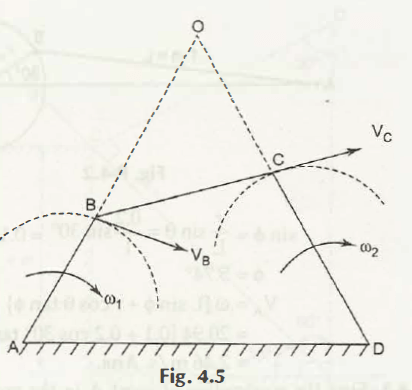Consider the Fig 4.5 that shows a four bar mechanism,Here,

Velocity at point B, VB = 1. AB = 0. BO

Or,

0 = 1. AB/ BO

Velocity at point C, VC = 2. CD = 0. CO

Or,

0 = 2. CD/ CO

Using the above two equations, we get the following ratio-

1/ 2 = CD/ AB . BO/ CO

Links of Next Mechanical Engineering Topics:-### Customer Reviews

My Homework Help
Rated 5.0 out of 5 based on 510 customer reviews at